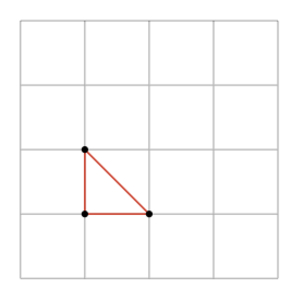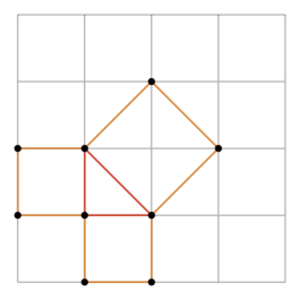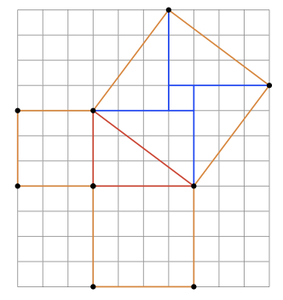# Sizing up Squares

Alignments to Content Standards: 8.G.B

1. Below is a picture of a right triangle on a square grid:In the next picture, quadrilaterals have been built on each side of the triangle:1. Explain why the quadrilateral sharing one side with the hypotenuse of the triangle is a square.
2. Find the areas of the three squares in the picture.
3. Is the sum of the areas of the squares on the legs equal to the area of the square on the hypotenuse?
2. Below is a second right triangle, with quadrilaterals built on the three sides of the triangle.1. What are the areas of the two squares built on the legs of the triangle?
2. Find the area of the quadrilateral sharing one side with the hypotenuse of the triangle.
3. The quadrilateral sharing one side with the hypotenuse is a square. Is its area equal to the sum of the areas of the squares built on the triangle legs?

## IM Commentary

The goal of this task is for students to check that the Pythagorean Theorem holds for two specific examples. Although the work of this task does not provide a proof for the full Pythagorean Theorem, it prepares students for the area calculations they will need to make as well as the difficulty of showing that a quadrilateral in the plane is a square.

For the right isosceles triangle, students can find the area of the three squares built on the sides of the triangle and verify that that the sum of the areas of the smaller squares is equal to the area of the largest square. One special feature of this right triangle is that students can produce a good argument for why the quadrilateral with the hypotenuse as one edge is a square. In the second example, this verification would be challenging and so students are allowed to assume that this quadrilateral is in fact a square. If the teacher wishes to pursue this aspect of the task in greater depth, several options are available:

• Students can investigate the symmetries of the quadrilateral using geometry software or patty paper.
• Students can see via rigid motions that the sides of the quadrilateral are congruent: this can be done via successive reflections of the coordinate grid or via rotations if students can identify the center of a 90 degree rotation which preserves the quadrilateral.
• Students can use the fact that the sum of the angles in a triangle are 180 degrees to show (using either of the pictures in the solution to (b)) that the four angles in the quadrilateral are right angles.

In modern times, the Pythagorean theorem is often stated in algebraic form: $a^2 + b^2 = c^2$ where $a$, $b$, and $c$ are the lengths of the sides of a right triangle, with $c$ being the length of the hypotenuse. In this task, the result is presented for two specific triangles in geometric form representative of the ancient Greek geometers' way of thinking.

## Solution

1.

1. We will first check that all four sides of the quadrilateral are congruent and then show that it has four right angles. The squares in the coordinate grid are all congruent with side length of one unit. This means that the diagonals of the coordinate grid squares are all congruent. All four sides of the quadrilateral built on the hypotenuse are diagonals of coordinate grid squares so they are all congruent.

Next we check that the four angles are right angles. Each angle of the quadrilateral is made up of two base angles of right isosceles triangles. These each measure 45 degrees so the four angles of the quadrilateral all measure 90 degrees and it is a square.

2. The squares built on the triangle legs are unit squares in the coordinate grid so they each have an area of 1 square unit. The coordinate grid lines divide the square on the hypotenuse into four half unit-squares, and so its area is 2 square units.
3. The sum of the areas of the squares built on the triangle's legs is 2 square units which is the same as the area of the square built on the triangle's hypotenuse.
2.

1. The square with side length 3 has an area of 9 square units while the square with side length 4 has an area of 16 square units.
2. There are many ways of doing this and two are shown here. All areas are calculated, as in part (a) above, in terms of square units where one square unit is a one unit by one unit square in the coordinate grid. The first method for calculating area decomposes the quadrilateral into right triangles and a square while the second method subtracts right triangles from a larger square.In the picture above, the quadrilateral on the triangle's hypotenuse is divided into 4 right triangles, each measuring 3 units by 4 units and a one unit by unit square. this means that the quadrilateral has an area of 25 square units.In this second picture the quadrilateral on the triangle's hypotenuse has been surrounded by a larger square measuring 7 units by 7 units. Four triangles, each measuring 3 by 4 units need to be removed to obtain the quadrilateral and so the area of the quadrilateral is 49 - 4$\times$6 or 25 square units.

3. The sum of the areas of the squares built on the triangle's legs is 9+16=25 square units which is the same as the area of the square built on the triangle's hypotenuse.